Dijkstra's Algorithm - Shortest Path

Posted on May 22nd, 2010
Note - This is not my area of expertise but I am very much interested in it and I welcome any corrections

Outline

This post will cover the basics of Dijksta's shortest path algorithm and how it can apply to path finding for game development. It is my opinion that understanding this algorithm will aid in understanding more complex AI algorithms, such as A*. This post is aimed more towards developers starting out in game development or those curious about Dijkstra's algorithm, but this will be a somewhat simplification of it and discuss mainly the concepts.

Introduction

What’s an algorithm?
An algorithm is basically a system for solving a problem. For us humans, looking at a 2d grid with many objects we can easily tell which path the character should take to reach his or her goal without thinking much about it. What we want to try to do is translate those semi-subconscious mental steps to a list of steps that anyone (or a computer) can repeat to get the same answer every time.

Finding the shortest route from one object to another when developing game AI is a very common problem and many solutions exist. At least in 2d grid / tile based games, perhaps the most common one is A*, with Dijkstra's being also quite good. Depending on the complexity of the game, Dijkstra's algorithm can be nearly as fast as A*, with some tweaking. A* is generally a better implementation, but can be slightly complex, so I'm going to discuss the fundamentals of Dijkstra's algorithm and in later posts talk about others, such as A*.

I'll be using the word graph here a lot, and it may not be immediately obvious how this translates to game dev, but you can easily translate this to 2d grid or tile based maps.

Dijkstra’s Algorithm

Let's first define what exactly the problem is. Take this graph, for instance.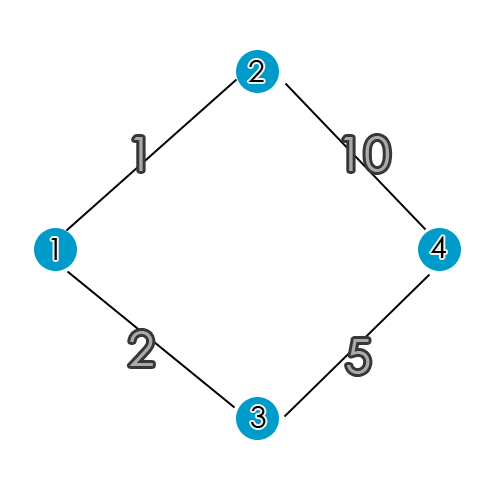For the purposes of this post, the blue circles represent "nodes" or "vertices" and the black lines are "edges" or "node paths". Each edge has a cost associated with it. For -this- image, the number in each node in this image is simply a label for the node, not the individual node cost.

Our problem is to find the most cost efficient route from Node1 to Node4. The numbers on the node paths represent the "cost" of going between nodes. The shortest path from Node1 to Node4 is to take Node1 to Node3 to Node4, as that is the path where the least cost is incurred.
Specifically, the cost to go from Node1 to Node3 is (2), plus the cost of Node3 to Node4 (5) is 7 (2 + 5).
Now, we can see that the alternative (Node1 to Node2 to Node4) is much more costly (it costs 11, versus our 7).
An important note - greedy algorithms aren't really effective here. A greedy algorithim would bascially find the cheapest local costs as it traverses the graph with the hopes that it would be globally optimum when it's done. Meaning, a greedy algorithm would basically just take the first low value it sees. In this case, the lower value is 1 but the next value is 10. If we were to simply just apply a greedy algorithm, we end up taking the more costly from Node1 to Node4.
Figuring out the best path to take with this graph is pretty easy for us to do mentally, as if you can add small numbers you can figure out the best path to take. For a small graph like the previous, it's quite easy. The goal is translate the steps we take in our mind to steps a computer follow.

Dijkstra's algorithm is an algorithm that will determine the best route to take, given a number of vertices (nodes) and edges (node paths). So, if we have a graph, if we follow Dijkstra's algorithm we can efficiently figure out the shortest route no matter how large the graph is.

Dijkstra's algorithm provides for us the shortest path from NodeA to NodeB.

This high level concept (not this algorithm specifically) is essentially how Google maps provides you directions. There are many thousands of vertices and edges, and when you ask for directions you typically want the shortest or least expensive route to and from your destinations.

So, how does this apply to game AI? Well, the correlation is quite strong. In a 2d grid or tile based map, there are many nodes (or tiles) and each tile can have a value associated with it (perhaps it is less expensive to walk across grass than it is to walk across broken bottles or lava).

You can set up your tiles so that each tile has a node path value associated with it, so if you put an non player character (NPC) in the map you can use Dijkstra's algorithm to compute the shortest path for the NPC to take to -ANY- tile in your map.

How it works

First we'll describe Dijsksta's algorithm in a few steps, and then expound on them furher:
Step 0.
Temporarily assign C(A) = 0 and C(x) = infinity for all other x.
C(A) means the Cost of A
C(x) means the current cost of getting to node x
Step 1.
Find the node x with the smallest temporary value of c(x).
If there are no temporary nodes or if c(x) = infinity, then stop.
Node x is now labeled as permanent. Node x is now labeled as the current node. C(x) and parent of x will not change again.
Step 2.
For each temporary node labeled vertex y adjacent to x, make the following comparison:
if c(x) + Wxy < c(y), then c(y) is changed to c(x) + Wxy
assign y to have parent x
Step 3.

Before diving into a little more tricky graph, we'll stick with the original graph introduced above. Let's get started.

Step 0.

Temporarily assign C(A) = 0 and C(x) = infinity for all other x. C(A) means the Cost of A C(x) means the current cost of getting to node x
The following graph has changed a little from the one shown above. The nodes no longer have labels, apart from our starting point NodeA and our goal NodeB.Legend
Orange line – path to parent node
Yellow arrow – points to the node’s parent
Green node cost text – node cost is permanent
White node cost test – node is temporary
Yellow highlight – Current node

We assign a cost of 0 to Node A and infinty to everything else. We're done with this step now.

Step 1.

Find the node x with the smallest temporary value of c(x).
If there are no temporary nodes or if c(x) = infinity, then stop.
Node x is now labeled as permanent. Node x is now labeled as the current node. C(x) and parent of x will not change again.
Since 0 is the lowest value, we set A as the current node and make it permanent.

Step 2.

For each temporary node labeled vertex y adjacent to x, make the following comparison:
if c(x) + Wxy < c(y), then
c(y) is changed to c(x) + Wxy
assign y to have parent x
There are two temporary nodes adjacent to our current node, so calcuate their cost values based on the current node's value + the cost of the adjacent node. Assign that value to the temporary node only if it's less than the value that's already there. So, to clarify:

The top node is adjacent to the current node and has a cost of infinity. 0 (the current node's value) + 1 (the cost associated with the temporary node) = 1, which is a less than infinity, so we change it's value from infinity to 1. This value is not yet permanent.

Now, do the same calucation for the next adjacent node. which is the bottom node. The value is 0 + 2 = 2, which is also less than infinity. To illustrate: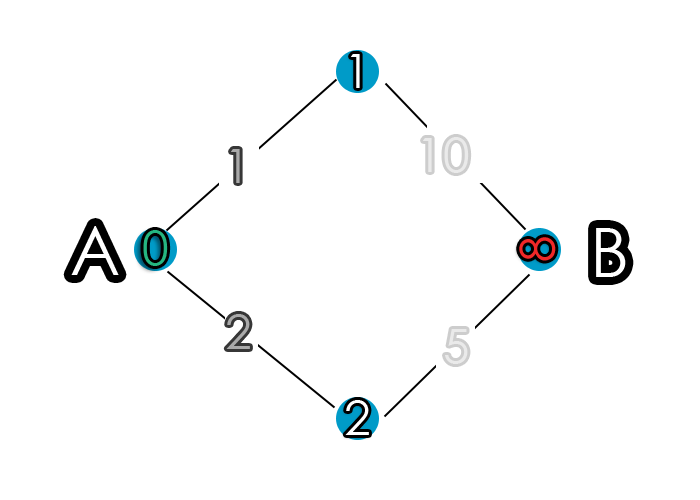So we now have looked at each temporary node adjacent to the current node, so we're done with this step.

Step 3.

So, let's go back to step 1. From this point forward, I'll be using the term iteration to describe our progression through the graph via Dijkstra's algorithm. The steps we previously took I'll refer to as iteration 0, so now when we return to step 1 we'll be at iteration 1.

Iteration 1

We’re back at the first step. It says look for the smallest temporary cost value and set it as permanent. We have two nodes to look at, the top node with cost 1 and the bottom node with cost 2.

The top node has a cost of 1, which is less than 2, so we set it as permanent and set it as our current node. We designate this by a yellow shadow in the image. Now, it is important to keep in mind that the bottom node still has a temporary cost assigned to it. This temporary cost is what allows the algorithm to find actual cheapest route – you’ll see in a second.

Step 1.

Find the cheapest node. Done, it’s set as permanent and our current node is this one. This node value will not change.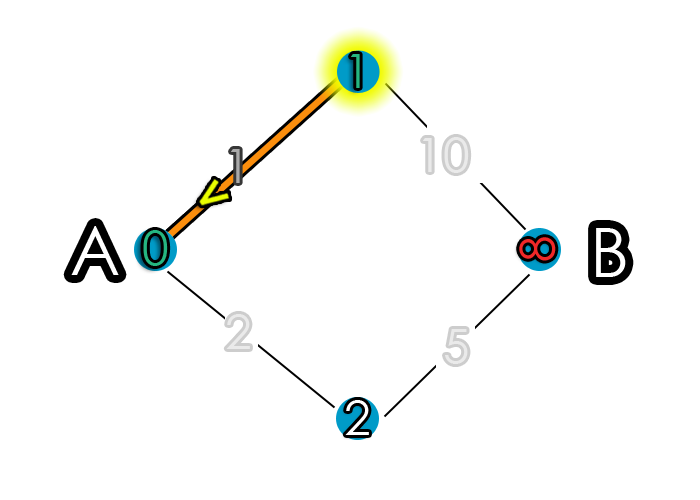The yellow highlight indictates the node we are currently on, and the green text means the node cost is permanent. The nodes with white text for their costs are temporary nodes.

Step 2.

Assign cost values. There is only one adjacent node to our current node. It's current value is infinity, which is less than 1 + 10, so we assign 11 to it's temporary cost value.This is not the shortest path from NodeA to NodeB, but that's fine. The algorithm traverses all nodes in the graph, so you get the shortest path from a node to any other node. You can see that the shortest path from NodeA to the top node is the line between NodeA and the top node - well, of course, you say, because that's the only possible path from NodeA to the top node. And you are right to say that, because it's true. But let's say we have a node above the top node (we'll call it Top2). The shortest path to that would from NodeA to the top node to node Top2. Even though our goal is to go from A to B, as a side effect we also get the shortest route to every other node. If that's a bit unclear, it should clear up after we go through the next iteration.

Done with step 2, let's continue to step 3.

Iteration 2

Ok, so now we look again at the temporary nodes to see which has the lowest value. Even though we calculated the temporary value of B to be 11, we are not done because that value might change (in this case, it will definitely change).

Step 1.

Pick the cheapest node and set it as our current node and make it permanent, and assign it its parent. We have two remaining temporary nodes with costs of 2 and 11. 2 is lower, so pick it and set it permanent and set it as our current node. Let’s take a look at the graph to elucidate a bit. So, out of 11 and 2, as we said, 2 is cheaper so pick it. We set this node’s value to be permanent and assign its parent is NodeA, demonstrated by the arrow.Step 2.

Assign cost values to temporary nodes adjacent to the current node. Again, like in the previous iteration, there is only one node to do a cost calculation on, as there is only one temporary node adjacent to the current node. This adjacent node is NodeB. So, we check to see if 2 + 5 < Node B’s temporary cost of 11. It is, so we change Node B from 11 to 7.Iteration 3

Almost done.

Step 1

Choose the cheapest temporary node value. There is only one temporary node remaining, so we pick it and set it as permanent, set it as our current node, and set it's parent.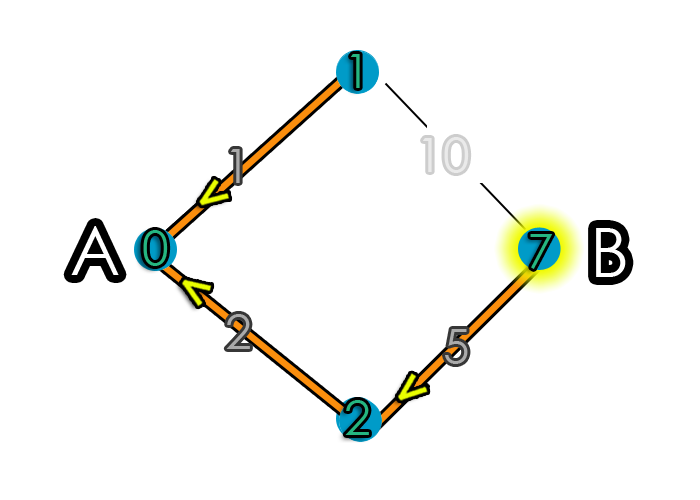Step 2.

Assign costs. There are no temporary nodes adjacent to Node B (there –are- permanent nodes, but we don’t check them).

Iteration 4

Step 1

Choose the cheapest temporary node. If none exists or c(x) = infinity, then stop. There are no more temporary nodes and no nodes have values of infinity, so we’re done. Algorithm has finished, and we have our shortest path from A to B, but also from that node to every other node in the graph. With such a small graph as this, it's not immediately obvious how powerful and useful this algorithim is.

So, on to a more complicated graph now.

More Complicated Graph

A is our starting point, and B is the ending point. Now, we could just as well apply this to a 2d tile based game where A could represent an NPC and B could represent the NPC's desired destination.

If you take a minute, you can probably find the least expensive route yourself. As mentioned earlier, it's fairly trivial for us to come up with the answer, what we need to do is figure out how to convey the steps we take to more extensible steps that can be repeated by a computer for any graph. For this graph, I won't be as thorough explaining every step, but the exact same process is applied. Instead, I'll just provide an example of a slightly more complex graph and what it would look like using Dijkstra's algorithm.

Step 0.

Temporarily assign C(A) = 0 and C(x) = infinity for all other x.
C(A) means the Cost of A
C(x) means the current cost of getting to node x
So what's this mean? Well, our start point is A so c(A) = 0 means assign A a cost of 0 and set the cost of x for every other node to infinity. Like the followingWe assign a cost of 0 to our starting node A and a cost of infinity to every other node. As before, none of these costs are permanent yet.

Step 1.

The node with the smallest temporary value is node A with a cost of 0. Therefore, we're going to make it permanent - meaning c(x) and the parent will not change.The 0 will not change now. If there are no temporary nodes, or if c(x) is infinity, the algorithm stops. Now, step 2.

Step 2.

Basically, we're going to look at all the nodes that are connected to the currently selected node and calculate the cost to get to them. If the cost of y is less than what it previously was, it will change - this will be discussed soon.

So, let's first calculate the cost to get to the adjacent nodes. The cost is based on the value of the current node code plus the edge (node path) cost. Right now, since this our first go, the cost our current node is at 0 since we haven't done any traversals.

So, let's start to figure out the c(x), the node costs.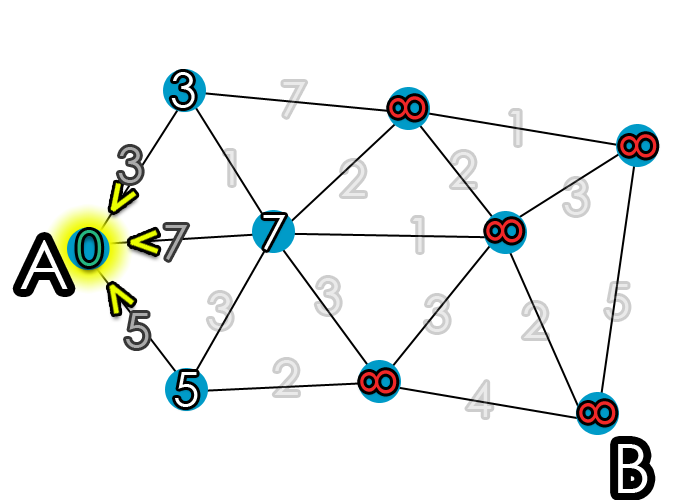Notice the yellow arrows. I'm using them to designate what node it got its cost from. Here, since there is only one possible parent node, they all point to the same place.

For the three nodes adjacent to A, we add the values of the edge and our current node (value of 0). So, the top node is 0 + 3 = 3, which is less than the current value (which is infinity), so we apply the value of 3 to the node. Then, the middle node 0 + 7 = 7, also less than infinity. Finally the bottom node has a value of 0 + 5 = 5, which is less than infinity. Therefore, the top node has a c(x) of 3, the middle a c(x) of 7, and the bottom a c(x) of 5.

Step 3.

As before, we just iteratively go through graph applying the same steps.

So, walking through this - as step 1 says:
We find node x with the smallest temporary value of c(x). So, out of the three temporary nodes with values 3, 5, and 7 that we just worked out, the smallest value is 3. We then make this node permanent.Now, this entire process just repeats itself over and over until there are no more temporary nodes.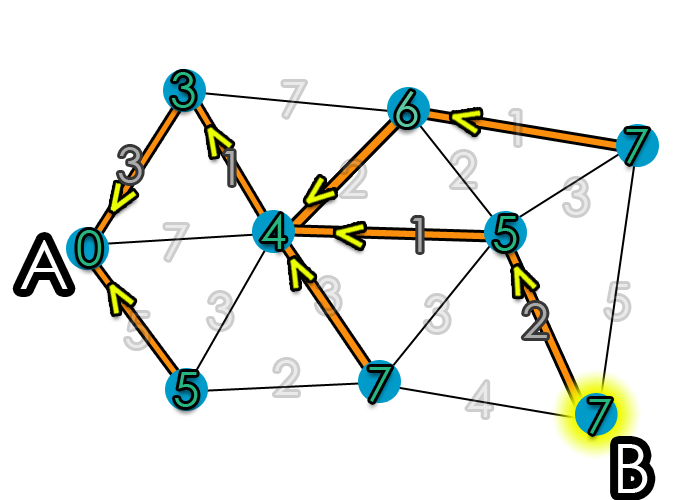And we're done. We have the shortest path a node to any other node (or vice versa). Pretty convenient.

Conclusion

Hopefully that explains a bit about how Dijkstra's Algorithm works. For game development, in particular overhead 2d tile based games, it is usually easier to implement Dijkstra's than A*, and not much worse performance wise.

Performance

How well does Dijsktra's algorithm perform? Well, in terms of big O notion it is O(n^2), which isn't that great. However, we must always consider what problem we're trying to solve, how big the desired input data is, and if we can make gains in other areas (e.g., caching). O(n^2) is like having a nested for loop.

Specifically, suppose G has n vertices and m edges. Going through the steps, Step 0 has time n. Step 1 is called, at the very most, n times. Finding the cheapest vertex takes at most n steps, so step 1 has an upper bound time of n^2. In Step 2, each edge / node path is examined, at most, twice. There, the upper bound time is 2m.

So, putting it all together, it's no worse than n^2 + 2m. Again, in "computer science" terms of algorithmic complexity, it is O(n^2); or: on the order of at most n^2 steps times a constant.

Conclusion

Better algorithms for NPC path finding certainly exist (see Amit's page on path finding algorithms, but in general Dijkstra's is often good enough and fairly easy to implement yourself. A very good explanation of implementation in python can be found at (written by the guy who wrote python) http://www.python.org/doc/essays/graphs.html